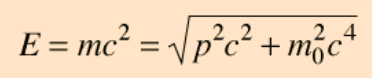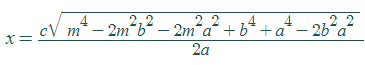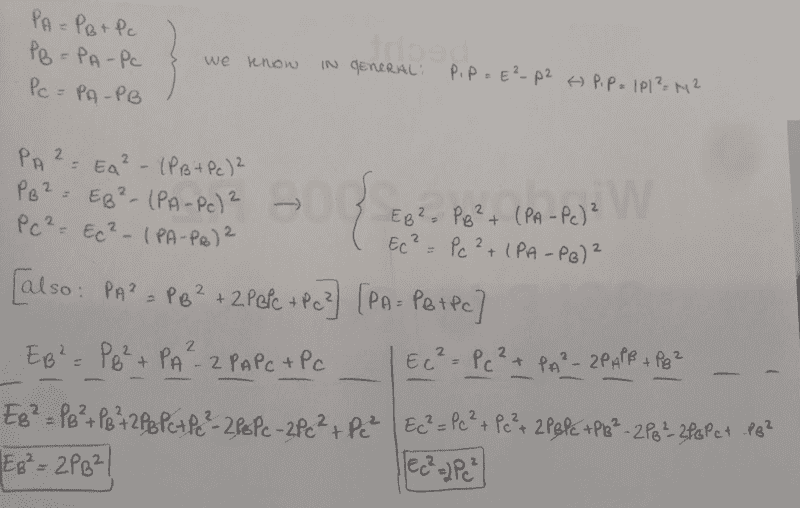# Momentum dealing with decay, special relativity

• Cocoleia
In summary, the conversation discusses the calculation of momentum for two daughter particles, B and C, after the decay of a mother particle, A. The solution involves conservation of momentum and energy, and can be simplified using relativistic invariants and dot products. The final result is that the momentum of B is equal to the momentum of C.

## Homework Statement

A particle A (mother particle) with a mass of mA decays to two particles B and C (daughter
particles) with mass values of respectively mB and mC. Calculate momentum of the two
daughter particles, pB and pC. (at first the mother particle is at rest)

## Homework Equations## The Attempt at a Solution

I would do conservation of momentum which would give me that pB=-pC since pA is 0 if it is at rest. then to do conservation of energy, before the decay E=mAc^2 and energy after the decary would be just replacing for the respective masses and momentums, and then solving for pB (or pC).

Using Symbolab:Where x is the momentum pB and a=mass of a, b=mass of b, m=mass of c and then c is the speed of light constant

Is this okay ? I am a bit unsure if I am missing something because of special relativity.

#### Attachments

You can check your result by making up a numerical example or two. For example, suppose m0A = 1000 MeV/c2, m0B = 700 MeV/c2 and m0C = 250 MeV/c2. Calculate the momentum of B and C after the decay using your result, then the energies of A, B, and C. Do the energies add up properly?

[added] Tip: if your energy unit is MeV, and your mass unit is MeV/c2, then the corresponding momentum unit is MeV/c.

Last edited:
In general, these sorts of problems are much easier to solve using relativistic invariants than with a straight-up, brute-force application of the quadratic formula. Let ##p_A##, ##p_B##, and ##p_C## denote the four-momenta of the three particles. Then ##p_A = p_B + p_C##. Rearranging this equation in two different ways gives: \begin{align*} p_B &= p_A - p_C \\ p_C &= p_A - p_B \end{align*} Now, take the relativistic dot product of each of these equations with itself. This should give simple expressions for the energies ##E_B## and ##E_C##. From there it's pretty straightforward to get the momenta.

EDIT: Just an additional hint--once you have the energies, you *could* use the invariant of each particle (e.g., ##E_B^2 - c^2 p_B^2 = m_B^2 c^4##) to directly solve for the momenta. But this leads to some gross algebra which can be avoided by relativistically squaring the first equation we looked at---i.e., ##p_A = p_B + p_C##---and substituting in the values you just found for ##E_B## and ##E_C##, remembering that we must have ##\vec{p}_B = -\vec{p}_C## (where over-arrows indicate three-momenta, not four-momenta).

In general, if you're using the quadratic formula, it's probable that you're missing a simpler solution.

Last edited:
VKint said:
In general, these sorts of problems are much easier to solve using relativistic invariants than with a straight-up, brute-force application of the quadratic formula. Let ##p_A##, ##p_B##, and ##p_C## denote the four-momenta of the three particles. Then ##p_A = p_B + p_C##. Rearranging this equation in two different ways gives: \begin{align*} p_B &= p_A - p_C \\ p_C &= p_A - p_B \end{align*} Now, take the relativistic dot product of each of these equations with itself. This should give simple expressions for the energies ##E_B## and ##E_C##. From there it's pretty straightforward to get the momenta.

EDIT: Just an additional hint--once you have the energies, you *could* use the invariant of each particle (e.g., ##E_B^2 - c^2 p_B^2 = m_B^2 c^4##) to directly solve for the momenta. But this leads to some gross algebra which can be avoided by relativistically squaring the first equation we looked at---i.e., ##p_A = p_B + p_C##---and substituting in the values you just found for ##E_B## and ##E_C##, remembering that we must have ##\vec{p}_B = -\vec{p}_C## (where over-arrows indicate three-momenta, not four-momenta).

In general, if you're using the quadratic formula, it's probable that you're missing a simpler solution.
Hi, thanks for the help. I'm just stuck at one point. I assume it very simple and I'm just not realizing it.

Here is what i have done so far:How do I somehow "finalize" the answer to get expressions for the momentum that don't involve the energy? I know I could use the definition but as you said it would involve some long algebra. I am confused with what you said about how to avoid the algebra.

If I do it, I get Pb=(sqrt2/2) mb, I am thinking this is wrong because the mass would be different for the two particles so there is no way that the momentum can be the same for the answer I found

#### Attachments

Last edited:
You're making it too complicated. Here's an example of what the kind of calculation you want to do. Let ##p_\text{A}##, ##p_\text{B}##, and ##p_\text{C}## denote the four-momenta of the respective particles. Conservation of momentum and energy gives you ##p_\text{A} = p_\text{B} + p_\text{C}##. Now suppose you square this equation. You get
$$p_\text{A}^2 = (p_\text{B}+p_\text{C})^2 = p_\text{B}^2 + 2 p_\text{B}\cdot p_\text{C} + p_\text{C}^2.$$ But ##p_\text{A}^2 = m_\text{A}^2##, ##p_\text{B}^2 = m_\text{B}^2##, and ##p_\text{C}^2 = m_\text{C}^2##, so we get
$$m_\text{A}^2 = m_\text{B}^2 + 2p_\text{B}\cdot p_\text{C} + m_\text{C}^2.$$ The only thing left involving energies and momenta is the dot product.

To solve the problem, use VKint's suggestion about rearranging the conservation-of-four-momentum equation and take advantage of the fact that ##\vec{p}_\text{A}=0## because A is at rest.

vela said:
You're making it too complicated. Here's an example of what the kind of calculation you want to do. Let ##p_\text{A}##, ##p_\text{B}##, and ##p_\text{C}## denote the four-momenta of the respective particles. Conservation of momentum and energy gives you ##p_\text{A} = p_\text{B} + p_\text{C}##. Now suppose you square this equation. You get
$$p_\text{A}^2 = (p_\text{B}+p_\text{C})^2 = p_\text{B}^2 + 2 p_\text{B}\cdot p_\text{C} + p_\text{C}^2.$$ But ##p_\text{A}^2 = m_\text{A}^2##, ##p_\text{B}^2 = m_\text{B}^2##, and ##p_\text{C}^2 = m_\text{C}^2##, so we get
$$m_\text{A}^2 = m_\text{B}^2 + 2p_\text{B}\cdot p_\text{C} + m_\text{C}^2.$$ The only thing left involving energies and momenta is the dot product.

To solve the problem, use VKint's suggestion about rearranging the conservation-of-four-momentum equation and take advantage of the fact that ##\vec{p}_\text{A}=0## because A is at rest.
But if I know that Pb^2=mb^2 then why do I have to do any other calculations ? I am just looking for these momentum , how did you find this.
Pb does not equal the mass, because Pb=-Pc and later on I am given an application where both particle do not have the same mass

Last edited:
Reading through your work in post #4, I'd strongly suggest you use the following convention: Let ##p## (normal typeface, without arrow) denote a relativistic four-momentum, and let ##\vec{p}## denote its corresponding *three-dimensional* momentum. I think you're confusing these two objects in your calculation.

Using this notation, the standard relativistic invariant is: ##p \cdot p = E^2 - c^2 | \vec{p} |^2 = m^2 c^4##. This is the fact Vela was referring to--however, it's standard practice to omit factors of ##c## (i.e., choose units in which ##c = 1##) for convenience, hence Vela's statement that "##p^2 = m^2##". Whichever version you use, this is the critical relationship that will allow you to solve this problem, and I don't see it showing up in your work.

For example: relativistically "squaring" (i.e., taking the relativistic dot product of both sides with themselves) the equation ##p_A = p_B + p_C## gives: \begin{align*} p_A \cdot p_A &= p_B \cdot p_B + 2 p_B \cdot p_C + p_C \cdot p_C \\ m_A^2 c^4 &= m_B^2 c^4 + 2 (E_B E_C - c^2 \vec{p}_B \cdot \vec{p}_C) + m_C^2 c^4 \end{align*} Now, we know that ##\vec{p}_B = -\vec{p}_C##, because ##\vec{p}_B + \vec{p}_C = \vec{p}_A## (conservation of momentum holds for three-momenta, too), and ##\vec{p}_A = 0##. This allows you to simplify the above equation a little bit--but there are still the energies ##E_B## and ##E_C## to deal with.

What I meant when I advised you to avoid "using the quadratic formula" was this: You could use the facts that ##E_B^2 - c^2 | \vec{p}_B |^2 = m_B^2 c^4## and ##E_C^2 - c^2 | \vec{p}_C |^2 = m_C^2 c^4## to solve for the energies in terms of the three-momenta, and substitute these into the above equation. This is, however, a *terrible* use of time and misses a much prettier, more efficient solution based on rearranging the original conservation-of-momentum relation.

Indeed, if we relativistically square ##p_B = p_A - p_C##, we get the following: \begin{align*} p_B \cdot p_B &= p_A \cdot p_A - 2 p_A \cdot p_C + p_C \cdot p_C \\ m_B^2 c^4 &= m_A^2 c^4 - 2(E_A E_C - c^2 \vec{p}_A \cdot \vec{p}_C) + m_C^2 c^4 \\ m_B^2 c^4 &= m_A^2 c^4 - 2E_A E_C + m_C^2 c^4 \end{align*} (The last line follows because ##\vec{p}_A = 0##.) Furthermore, since A is at rest, ##E_A = m_A c^2##. Substituting this into the above will allow you to solve for ##E_C## in terms of the masses, and squaring the equation ##p_C = p_A - p_B## will similarly allow you to solve for ##E_B##. Once you have these, you can just substitute the energies back into the first equation to solve for the three-momentum ##\vec{p}_B = -\vec{p}_C##.